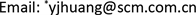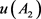﻿ 环氧乙烷检测仪的校准方法研究 Calibration of the Halogen Leak Detector

Instrumentation and Equipments
Vol. 07  No. 01 ( 2019 ), Article ID: 29357 , 5 pages
10.12677/IaE.2019.71008

Calibration of the Halogen Leak Detector

Zhaoqun Xie, Meng Zhang, Najun Jia, Zichen Huang, Junbin Xu, Yanjie Huang*, Jinyan Zhou

Guangdong Provincial Key Laboratory of Modern Geometric and Mechanical Metrology Technology, Guangdong Provincial Institute of Metrology, Guangzhou GuangdongReceived: Feb. 24th, 2019; accepted: Mar. 13th, 2019; published: Mar. 21st, 2019ABSTRACT

Based on the principle and application of the ethylene oxide detector, this paper puts forward the measurement calibration method of the ethylene oxide detector, and discusses the measurement characteristics, calibration conditions, calibration items and calibration methods. The calibration items including appearance inspection, indication error, repeatability and response time are discussed in detail. The calibration method and result evaluation of the ethylene oxide detector described in this paper will provide a good guidance for calibration of related instruments, and ensure the accuracy and comparability of future measurement results and traceability of quantities.

Keywords:Epoxyethane, Detector, Uncertainty, Indication Error1. 引言

2. 计量特性

2.1. 测量范围

(0~1000) µmol/mol。

2.2. 示值误差

2.3. 重复性

2.4. 相应时间

3. 校准条件

3.1. 校准环境

1) 环境温度：(0~40)℃；

2) 相对湿度：≤70% RH；

3) 大气压力：(70.0~106.0) kPa，必须在有良好的通风条件下进行。

3.2. 校准用设备

1) 气体标准物质，氮中环氧乙烷气体标准物质或空气中环氧乙烷气体标准物质(以下简称标准气体)，其扩展不确定度应不大于3% (k = 2)；

2) 零点气：高纯氮气或干净空气；

3) 流量计：(0~1) L/min，准确度级别不低于4级；

4) 秒表：准确度为0.1秒。

4. 校准项目和校准方法

4.1. 外观检查

4.2. 示值误差

${\Delta }_{e}=\frac{\stackrel{¯}{A}-{A}_{s}}{{A}_{s}}×100%$ (1)

${\Delta }_{e}=\stackrel{¯}{A}-{A}_{s}$ (2)

4.3. 重复性

$S=\frac{1}{\stackrel{¯}{A}}\sqrt{\frac{\underset{i=1}{\overset{6}{\sum }}{\left({A}_{i}-\stackrel{¯}{A}\right)}^{2}}{5}}$ (3)

4.4. 响应时间

5. 环氧乙烷检测仪测量结果的测量不确定度的评定

5.1. 数学模型

${u}_{c}^{2}\left(\Delta \right)={c}_{1}^{2}{u}^{2}\left(\stackrel{¯}{A}\right)+{c}_{2}^{2}{u}^{2}\left({A}_{s}\right)={u}^{2}\left(\stackrel{¯}{A}\right)+{u}^{2}\left({A}_{s}\right)$ (4)

5.2. 不确定度的来源

5.3. 分量标准不确定度分析

1) 由气体标准物质引入的标准不确定度 $u\left({A}_{s}\right)$ 用B类标准不确定度评定，由标准物质证书可知，标准气体的相对扩展不确定度U95 = 2%，包含因子k = 2，所以 $u\left({A}_{s}\right)=50\text{\hspace{0.17em}}\text{μmol}/\text{mol}×2%/2=0.5\text{\hspace{0.17em}}\text{μmol}/\text{mol}$

2) 被测仪器重复性引入的标准不确定度 $u\left({A}_{1}\right)$ 用A类标准不确定度评定。将50 µmol/mol标准气体通入被测仪器，待示值稳定后，重复测量10次，分别读取测量值，其标准偏差s ≈ 1.1 µmol/mol，而实际校准测量中，重复次数3次，故 $u\left({A}_{1}\right)=1.1\text{\hspace{0.17em}}\text{μmol}/\text{mol}/\sqrt{3}=0.6\text{\hspace{0.17em}}\text{μmol}/\text{mol}$

3) 被测仪器读数(分辨力)引入的标准不确定度用B类标准不确定度评定。被测仪器的分度值为1 µmol/mol，半宽度0.5 µmol/mol，按均匀分布得： $u\left({A}_{2}\right)=0.5\text{\hspace{0.17em}}\text{μmol}/\text{mol}/\sqrt{3}=0.03\text{\hspace{0.17em}}\text{μmol}/\text{mol}$

5.4. 合成标准不确定度

${u}_{c}\left(\Delta A\right)=\sqrt{{u}^{2}\left({A}_{s}\right)+{u}^{2}\left({A}_{1}\right)+{u}^{2}\left({A}_{2}\right)}\approx 0.\text{84}\text{\hspace{0.17em}}\text{μmol}/\text{mol}$ (5)

5.5. 扩展标准不确定度计算

$U=k{u}_{c}\left(\Delta A\right)=1.7\text{\hspace{0.17em}}\text{μmol}/\text{mol}$ (6)

6. 结论

Calibration of the Halogen Leak Detector[J]. 仪器与设备, 2019, 07(01): 53-57. https://doi.org/10.12677/IaE.2019.71008

1. 1. 刘晓勇, 戎伟丰, 阮小林, 等. 溶剂解吸-气相色谱法测定工作场所空气中环氧乙烷[J]. 中国卫生检验杂志, 2016(4): 469-471.

2. 2. 郭春梅. 环氧乙烷检测报警仪校准方法的研究[J]. 计量技术, 2015(1): 57-59.

3. 3. 郑涤新, 刘星, 耿媛媛. 顶空气相色谱法测定医疗器械成品库空气中的环氧乙烷[J]. 分析仪器, 2011, 2011(1): 29-31.

4. 4. 张敬轩, 李挥, 蔡立鹏, 等. 顶空进样-气相色谱-质谱法检测清洁类化妆品中环氧乙烷和环氧丙烷残留量[J]. 分析化学, 2013, 41(8): 1293-1294.

5. 5. 蔡建华, 王晓艳, 陈岚, 等. JJG915-2008 一样换弹检测报警器检定规程[S]. 北京: 中国质检出版社, 2009.

6. 6. 杨铸, 姜传胜, 朱刚, 等. GB 12358-2006作业场所环境气体检测报警仪通用技术要求[S]. 北京: 中国标准出版社, 2007.

7. 7. 叶德培, 施昌彦, 金华彰, 等. JJF 1001-2011 通用计量术语及定义[S]. 北京: 中国质检出版社, 2012.

8. 8. 施昌彦, 叶德培, 陈明华, 等. JJF 1094-2002 测量仪器特性评定[S]. 北京: 中国质检出版社, 2003.

9. 9. 叶德培, 赵峰, 施昌彦, 等. JJF 1059.1-2012 测量不确定度评定与表示[S]. 北京: 中国标准出版社, 2013.